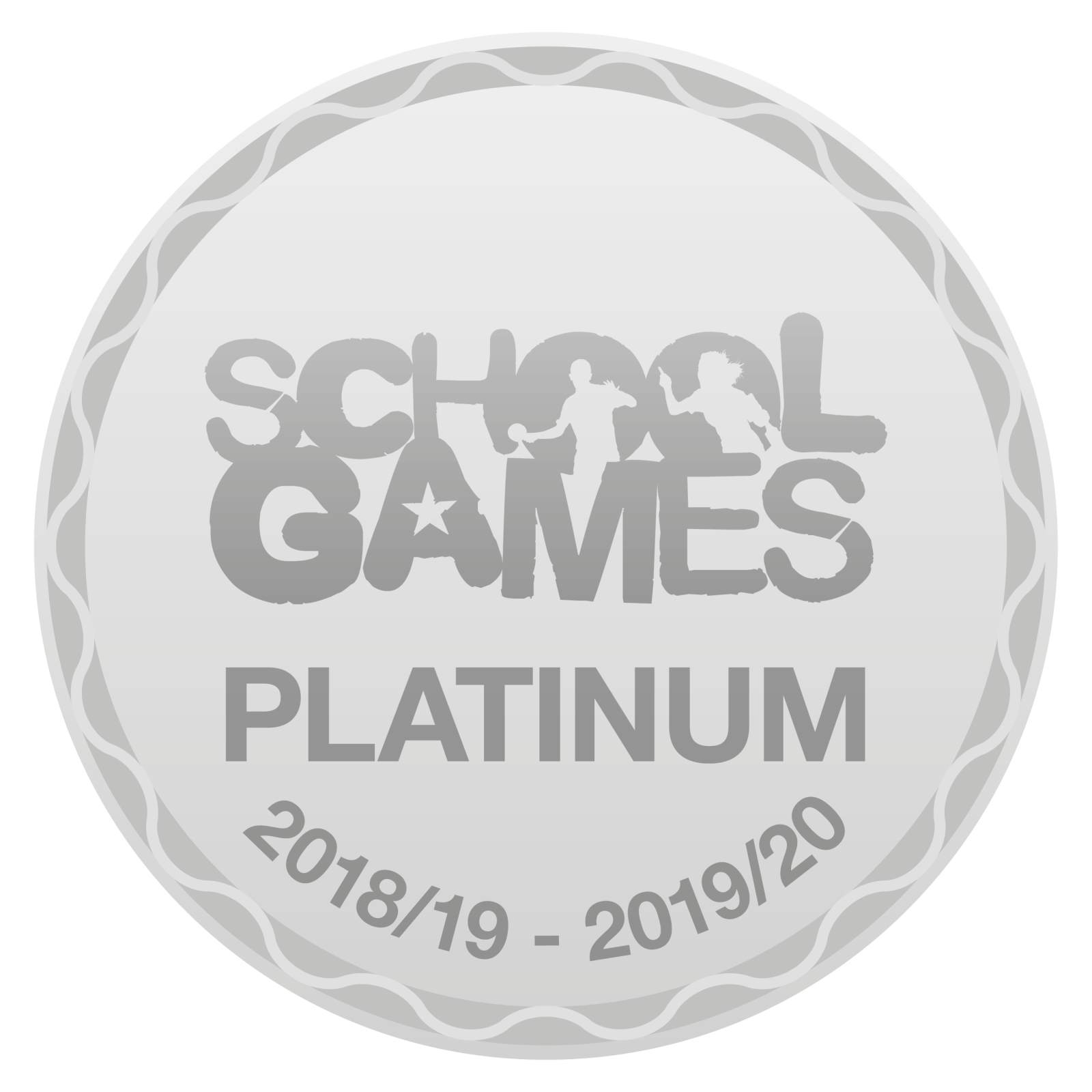Useful Links# Curriculum Lead - Mrs Hetherington

“Many people think that some students can work to high levels and some cannot because of the brains they are born with, but this idea has been resoundingly disproved. Study after study has shown the incredible capacity of brains to grow and change within a remarkably short period of time”.

Professor Jo Boaler, Stanford University- Growth Mindset Maths(youcubed)

Mathematics introduces children to concepts, skills and thinking strategies that are essential in everyday life and support learning across the curriculum.  It helps children make sense of the numbers, patterns and shapes they see in the world around them, offers ways of handling data in an increasingly digital world and makes a crucial contribution to their development as successful learners.

Children enjoy using mathematics to solve a problem, especially when it leads to an unexpected discovery or new connections.  As their confidence grows, they look for patterns, use logical reasoning, suggest solutions and try out different approaches to problems.

Mathematics offers children a powerful way of communicating.  They learn to explore and explain their ideas using symbols, diagrams and spoken and written language.  They start to discover how mathematics has developed over time and contributes to our economy, society and culture. Studying mathematics stimulates curiosity, fosters creativity and equips children with the skills they need in life beyond school.

Mastery Approach

New Pasture Lane staff care passionately about maths and have adopted (with the help of ‘Hamilton trust’ and ‘White Rose’) a ‘teaching for mastery’ approach, which has proven to have a positive impact upon children’s learning. Staff use a variety of sources to help them on their journey. Each term the children are assessed using a suite of standardised maths tests (PUMA), which then enables the school to track progress, predict future performance and ‘fill in’ any knowledge gaps.

Furthermore as the children progress through the school, they are constantly challenged and provided with many opportunities to express themselves. Therefore they may find themselves participating in Tute interactive online lessons, the UK’s ‘Primary Maths Challenge’ or even competing in the World Class Maths Arena.

Y5/6 Pupils who completed the Primary Maths Challenge..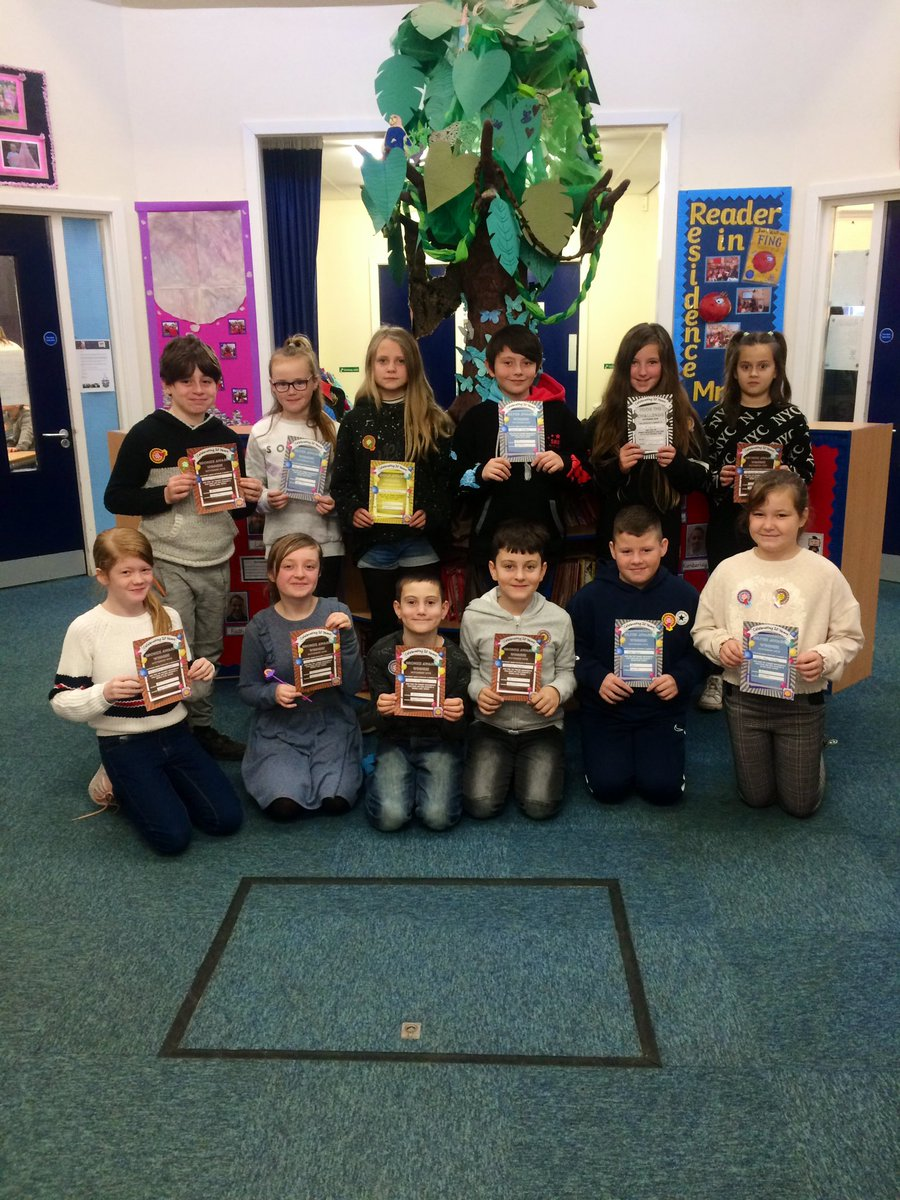In Early Years Foundation Stage children should be taught to:

• Have a deep understanding of number to 10, including the composition of each number;
• Subitise (recognise quantities without counting) up to 5
• Automatically recall (without reference to rhymes, counting or other aids) number bonds up to 5 (including subtraction facts) and some number bonds to 10, including double facts
• Verbally count beyond 20, recognising the pattern of the counting system
• Compare quantities up to 10 in different contexts, recognising when one quantity is greater than, less than or the same as the other quantity
• Explore and represent patterns within numbers up to 10, including evens and odds, double facts and how quantities can be distributed equally (Taken from: Statutory framework for the early years foundation stage 1 September 2021)

In Year 1 pupils should be taught to:

• count to and across 100, forwards and backwards, beginning with 0 or 1, or from any given number
• count, read and write numbers to 100 in numerals; count in multiples of 2s, 5s and 10s
• given a number, identify 1 more and 1 less
• identify and represent numbers using objects and pictorial representations including the number line, and use the language of: equal to, more than, less than (fewer), most, least
• read and write numbers from 1 to 20 in numerals and words
• read, write and interpret mathematical statements involving addition (+), subtraction (−) and equals (=) signs
• represent and use number bonds and related subtraction facts within 20
• add and subtract one-digit and two-digit numbers to 20, including 0
• solve one-step problems that involve addition and subtraction, using concrete objects and pictorial representations, and missing number problems such as 7 = ? − 9
• solve one-step problems involving multiplication and division, by calculating the answer using concrete objects, pictorial representations and arrays with the support of the teacher
• recognise, find and name a half as 1 of 2 equal parts of an object, shape or quantity
• recognise, find and name a quarter as 1 of 4 equal parts of an object, shape or quantity
• compare, describe and solve practical problems for:
• lengths and heights [for example, long/short, longer/shorter, tall/short, double/half]
• mass/weight [for example, heavy/light, heavier than, lighter than]
• capacity and volume [for example, full/empty, more than, less than, half, half full, quarter]
• time [for example, quicker, slower, earlier, later]
• measure and begin to record the following:
• lengths and heights
• mass/weight
• capacity and volume
• time (hours, minutes, seconds)
• recognise and know the value of different denominations of coins and notes
• sequence events in chronological order using language [for example, before and after, next, first, today, yesterday, tomorrow, morning, afternoon and evening]
• recognise and use language relating to dates, including days of the week, weeks, months and years
• tell the time to the hour and half past the hour and draw the hands on a clock face to show these times
• recognise and name common 2-D and 3-D shapes, including:
• 2-D shapes [for example, rectangles (including squares), circles and triangles]
• 3-D shapes [for example, cuboids (including cubes), pyramids and spheres]
• describe position, direction and movement, including whole, half, quarter and three-quarter turns

In Year 2 pupils should be taught to:

• count in steps of 2, 3, and 5 from 0, and in 10s from any number, forward and backward
• recognise the place value of each digit in a two-digit number (10s, 1s)
• identify, represent and estimate numbers using different representations, including the number line
• compare and order numbers from 0 up to 100; use <, > and = signs
• read and write numbers to at least 100 in numerals and in words
• use place value and number facts to solve problems
• solve problems with addition and subtraction:
• using concrete objects and pictorial representations, including those involving numbers, quantities and measures
• applying their increasing knowledge of mental and written methods
• recall and use addition and subtraction facts to 20 fluently, and derive and use related facts up to 100
• add and subtract numbers using concrete objects, pictorial representations, and mentally, including:
• a two-digit number and 1s
• a two-digit number and 10s
• 2 two-digit numbers
• show that addition of 2 numbers can be done in any order (commutative) and subtraction of 1 number from another cannot
• recognise and use the inverse relationship between addition and subtraction and use this to check calculations and solve missing number problems
• recall and use multiplication and division facts for the 2, 5 and 10 multiplication tables, including recognising odd and even numbers
• calculate mathematical statements for multiplication and division within the multiplication tables and write them using the multiplication (×), division (÷) and equals (=) signs
• show that multiplication of 2 numbers can be done in any order (commutative) and division of 1 number by another cannot
• solve problems involving multiplication and division, using materials, arrays, repeated addition, mental methods, and multiplication and division facts, including problems in contexts
• recognise, find, name and write fractions ,  ,  and  of a length, shape, set of objects or quantity
• write simple fractions, for example of 6 = 3 and recognise the equivalence of  and
• choose and use appropriate standard units to estimate and measure length/height in any direction (m/cm); mass (kg/g); temperature (°C); capacity (litres/ml) to the nearest appropriate unit, using rulers, scales, thermometers and measuring vessels
• compare and order lengths, mass, volume/capacity and record the results using >, < and =
• recognise and use symbols for pounds (£) and pence (p); combine amounts to make a particular value
• find different combinations of coins that equal the same amounts of money
• solve simple problems in a practical context involving addition and subtraction of money of the same unit, including giving change
• compare and sequence intervals of time
• tell and write the time to five minutes, including quarter past/to the hour and draw the hands on a clock face to show these times
• know the number of minutes in an hour and the number of hours in a day
• identify and describe the properties of 2-D shapes, including the number of sides, and line symmetry in a vertical line
• identify and describe the properties of 3-D shapes, including the number of edges, vertices and faces
• identify 2-D shapes on the surface of 3-D shapes, [for example, a circle on a cylinder and a triangle on a pyramid]
• compare and sort common 2-D and 3-D shapes and everyday objects
• order and arrange combinations of mathematical objects in patterns and sequences
• use mathematical vocabulary to describe position, direction and movement, including movement in a straight line and distinguishing between rotation as a turn and in terms of right angles for quarter, half and three-quarter turns (clockwise and anti-clockwise)
• interpret and construct simple pictograms, tally charts, block diagrams and tables
• ask and answer simple questions by counting the number of objects in each category and sorting the categories by quantity

In Year 3 pupils should be taught to:

• count from 0 in multiples of 4, 8, 50 and 100; find 10 or 100 more or less than a given number
• recognise the place value of each digit in a 3-digit number (100s, 10s, 1s)
• compare and order numbers up to 1,000
• identify, represent and estimate numbers using different representations
• read and write numbers up to 1,000 in numerals and in words
• solve number problems and practical problems involving these ideas
• add and subtract numbers mentally, including:
• a three-digit number and 1s
• a three-digit number and 10s
• a three-digit number and 100s
• add and subtract numbers with up to 3 digits, using formal written methods of columnar addition and subtraction
• estimate the answer to a calculation and use inverse operations to check answers
• solve problems, including missing number problems, using number facts, place value, and more complex addition and subtraction
• recall and use multiplication and division facts for the 3, 4 and 8 multiplication tables
• write and calculate mathematical statements for multiplication and division using the multiplication tables that they know, including for two-digit numbers times one-digit numbers, using mental and progressing to formal written methods
• solve problems, including missing number problems, involving multiplication and division, including positive integer scaling problems and correspondence problems in which n objects are connected to m objects
• recognise, find and write fractions of a discrete set of objects: unit fractions and non-unit fractions with small denominators
• recognise and use fractions as numbers: unit fractions and non-unit fractions with small denominators
• recognise and show, using diagrams, equivalent fractions with small denominators
• add and subtract fractions with the same denominator within one whole [for example, +  =  ]
• compare and order unit fractions, and fractions with the same denominators
• solve problems that involve all of the above
• measure, compare, add and subtract: lengths (m/cm/mm); mass (kg/g); volume/capacity (l/ml)
• measure the perimeter of simple 2-D shapes
• add and subtract amounts of money to give change, using both £ and p in practical contexts
• tell and write the time from an analogue clock, including using Roman numerals from I to XII, and 12-hour and 24-hour clocks
• estimate and read time with increasing accuracy to the nearest minute; record and compare time in terms of seconds, minutes and hours; use vocabulary such as o’clock, am/pm, morning, afternoon, noon and midnight
• know the number of seconds in a minute and the number of days in each month, year and leap year
• compare durations of events [for example, to calculate the time taken by particular events or tasks]
• draw 2-D shapes and make 3-D shapes using modelling materials; recognise 3-D shapes in different orientations and describe them
• recognise angles as a property of shape or a description of a turn
• identify right angles, recognise that 2 right angles make a half-turn, 3 make three-quarters of a turn and 4 a complete turn; identify whether angles are greater than or less than a right angle
• identify horizontal and vertical lines and pairs of perpendicular and parallel lines
• solve one-step and two-step questions [for example ‘How many more?’ and ‘How many fewer?’] using information presented in scaled bar charts and pictograms and tables

In Year 4 pupils should be taught to:

• count in multiples of 6, 7, 9, 25 and 1,000
• find 1,000 more or less than a given number
• count backwards through 0 to include negative numbers
• recognise the place value of each digit in a four-digit number (1,000s, 100s, 10s, and 1s)
• order and compare numbers beyond 1,000
• identify, represent and estimate numbers using different representations
• round any number to the nearest 10, 100 or 1,000
• solve number and practical problems that involve all of the above and with increasingly large positive numbers
• read Roman numerals to 100 (I to C) and know that over time, the numeral system changed to include the concept of 0 and place value
• add and subtract numbers with up to 4 digits using the formal written methods of columnar addition and subtraction where appropriate
• estimate and use inverse operations to check answers to a calculation
• solve addition and subtraction two-step problems in contexts, deciding which operations and methods to use and why
• recall multiplication and division facts for multiplication tables up to 12 × 12
• use place value, known and derived facts to multiply and divide mentally, including: multiplying by 0 and 1; dividing by 1; multiplying together 3 numbers
• recognise and use factor pairs and commutativity in mental calculations
• multiply two-digit and three-digit numbers by a one-digit number using formal written layout
• solve problems involving multiplying and adding, including using the distributive law to multiply two-digit numbers by 1 digit, integer scaling problems and harder correspondence problems such as n objects are connected to m objects
• recognise and show, using diagrams, families of common equivalent fractions
• count up and down in hundredths; recognise that hundredths arise when dividing an object by 100 and dividing tenths by 10
• solve problems involving increasingly harder fractions to calculate quantities, and fractions to divide quantities, including non-unit fractions where the answer is a whole number
• add and subtract fractions with the same denominator
• recognise and write decimal equivalents of any number of tenths or hundreds
• recognise and write decimal equivalents to ,  ,
• find the effect of dividing a one- or two-digit number by 10 and 100, identifying the value of the digits in the answer as ones, tenths and hundredths
• round decimals with 1 decimal place to the nearest whole number
• compare numbers with the same number of decimal places up to 2 decimal places
• solve simple measure and money problems involving fractions and decimals to 2 decimal places
• convert between different units of measure [for example, kilometre to metre; hour to minute]
• measure and calculate the perimeter of a rectilinear figure (including squares) in centimetres and metres
• find the area of rectilinear shapes by counting squares
• estimate, compare and calculate different measures, including money in pounds and pence
• read, write and convert time between analogue and digital 12- and 24-hour clocks
• solve problems involving converting from hours to minutes, minutes to seconds, years to months, weeks to days
• compare and classify geometric shapes, including quadrilaterals and triangles, based on their properties and sizes
• identify acute and obtuse angles and compare and order angles up to 2 right angles by size
• identify lines of symmetry in 2-D shapes presented in different orientations
• complete a simple symmetric figure with respect to a specific line of symmetry
• describe positions on a 2-D grid as coordinates in the first quadrant
• describe movements between positions as translations of a given unit to the left/right and up/down
• plot specified points and draw sides to complete a given polygon
• interpret and present discrete and continuous data using appropriate graphical methods, including bar charts and time graphs
• solve comparison, sum and difference problems using information presented in bar charts, pictograms, tables and other graphs

In Year 5 pupils should be taught to:

• read, write, order and compare numbers to at least 1,000,000 and determine the value of each digit
• count forwards or backwards in steps of powers of 10 for any given number up to 1,000,000
• interpret negative numbers in context, count forwards and backwards with positive and negative whole numbers, including through 0
• round any number up to 1,000,000 to the nearest 10, 100, 1,000, 10,000 and 100,000
• solve number problems and practical problems that involve all of the above
• read Roman numerals to 1,000 (M) and recognise years written in Roman numerals
• add and subtract whole numbers with more than 4 digits, including using formal written methods (columnar addition and subtraction)
• add and subtract numbers mentally with increasingly large numbers
• use rounding to check answers to calculations and determine, in the context of a problem, levels of accuracy
• solve addition and subtraction multi-step problems in contexts, deciding which operations and methods to use and why
• identify multiples and factors, including finding all factor pairs of a number, and common factors of 2 numbers
• know and use the vocabulary of prime numbers, prime factors and composite (non-prime) numbers
• establish whether a number up to 100 is prime and recall prime numbers up to 19
• multiply numbers up to 4 digits by a one- or two-digit number using a formal written method, including long multiplication for two-digit numbers
• multiply and divide numbers mentally, drawing upon known facts
• divide numbers up to 4 digits by a one-digit number using the formal written method of short division and interpret remainders appropriately for the context
• multiply and divide whole numbers and those involving decimals by 10, 100 and 1,000
• recognise and use square numbers and cube numbers, and the notation for squared (²) and cubed (³)
• solve problems involving multiplication and division, including using their knowledge of factors and multiples, squares and cubes
• solve problems involving addition, subtraction, multiplication and division and a combination of these, including understanding the meaning of the equals sign
• solve problems involving multiplication and division, including scaling by simple fractions and problems involving simple rates
• compare and order fractions whose denominators are all multiples of the same number
• identify, name and write equivalent fractions of a given fraction, represented visually, including tenths and hundredths
• recognise mixed numbers and improper fractions and convert from one form to the other and write mathematical statements > 1 as a mixed number [for example, +  =  = 1  ]
• add and subtract fractions with the same denominator, and denominators that are multiples of the same number
• multiply proper fractions and mixed numbers by whole numbers, supported by materials and diagrams
• read and write decimal numbers as fractions [for example, 0.71 = ]
• recognise and use thousandths and relate them to tenths, hundredths and decimal equivalents
• round decimals with 2 decimal places to the nearest whole number and to 1 decimal place
• read, write, order and compare numbers with up to 3 decimal places
• solve problems involving number up to 3 decimal places
• recognise the per cent symbol (%) and understand that per cent relates to ‘number of parts per 100’, and write percentages as a fraction with denominator 100, and as a decimal fraction
• solve problems which require knowing percentage and decimal equivalents of ,  ,  ,  ,  and those fractions with a denominator of a multiple of 10 or 25
• convert between different units of metric measure [for example, kilometre and metre; centimetre and metre; centimetre and millimetre; gram and kilogram; litre and millilitre]
• understand and use approximate equivalences between metric units and common imperial units such as inches, pounds and pints
• measure and calculate the perimeter of composite rectilinear shapes in centimetres and metres
• calculate and compare the area of rectangles (including squares), including using standard units, square centimetres (cm²) and square metres (m²), and estimate the area of irregular shapes
• estimate volume [for example, using 1 cm³ blocks to build cuboids (including cubes)] and capacity [for example, using water]
• solve problems involving converting between units of time
• use all four operations to solve problems involving measure [for example, length, mass, volume, money] using decimal notation, including scaling
• identify 3-D shapes, including cubes and other cuboids, from 2-D representations
• know angles are measured in degrees: estimate and compare acute, obtuse and reflex angles
• draw given angles, and measure them in degrees (°)
• identify:
• angles at a point and 1 whole turn (total 360°)
• angles at a point on a straight line and half a turn (total 180°)
• other multiples of 90°
• use the properties of rectangles to deduce related facts and find missing lengths and angles
• distinguish between regular and irregular polygons based on reasoning about equal sides and angles
• identify, describe and represent the position of a shape following a reflection or translation, using the appropriate language, and know that the shape has not changed
• solve comparison, sum and difference problems using information presented in a line graph
• complete, read and interpret information in tables, including timetables

In Year 6 pupils should be taught to:

• read, write, order and compare numbers up to 10,000,000 and determine the value of each digit
• round any whole number to a required degree of accuracy
• use negative numbers in context, and calculate intervals across 0
• solve number and practical problems that involve all of the above
• multiply multi-digit numbers up to 4 digits by a two-digit whole number using the formal written method of long multiplication
• divide numbers up to 4 digits by a two-digit whole number using the formal written method of long division, and interpret remainders as whole number remainders, fractions, or by rounding, as appropriate for the context
• divide numbers up to 4 digits by a two-digit number using the formal written method of short division where appropriate, interpreting remainders according to the context
• perform mental calculations, including with mixed operations and large numbers
• identify common factors, common multiples and prime numbers
• use their knowledge of the order of operations to carry out calculations involving the 4 operations
• solve addition and subtraction multi-step problems in contexts, deciding which operations and methods to use and why
• solve problems involving addition, subtraction, multiplication and division
• use estimation to check answers to calculations and determine, in the context of a problem, an appropriate degree of accuracy
• use common factors to simplify fractions; use common multiples to express fractions in the same denomination
• compare and order fractions, including fractions >1
• add and subtract fractions with different denominators and mixed numbers, using the concept of equivalent fractions
• multiply simple pairs of proper fractions, writing the answer in its simplest form [for example, ×  =  ]
• divide proper fractions by whole numbers [for example, ÷ 2 =  ]
• associate a fraction with division and calculate decimal fraction equivalents [for example, 0.375] for a simple fraction [for example, ]
• identify the value of each digit in numbers given to 3 decimal places and multiply and divide numbers by 10, 100 and 1,000 giving answers up to 3 decimal places
• multiply one-digit numbers with up to 2 decimal places by whole numbers
• use written division methods in cases where the answer has up to 2 decimal places
• solve problems which require answers to be rounded to specified degrees of accuracy
• recall and use equivalences between simple fractions, decimals and percentages, including in different contexts
• solve problems involving the relative sizes of 2 quantities where missing values can be found by using integer multiplication and division facts
• solve problems involving the calculation of percentages [for example, of measures and such as 15% of 360] and the use of percentages for comparison
• solve problems involving similar shapes where the scale factor is known or can be found
• solve problems involving unequal sharing and grouping using knowledge of fractions and multiples
• use simple formulae
• generate and describe linear number sequences
• express missing number problems algebraically
• find pairs of numbers that satisfy an equation with 2 unknowns
• enumerate possibilities of combinations of 2 variables
• solve problems involving the calculation and conversion of units of measure, using decimal notation up to 3 decimal places where appropriate
• use, read, write and convert between standard units, converting measurements of length, mass, volume and time from a smaller unit of measure to a larger unit, and vice versa, using decimal notation to up to 3 decimal places
• convert between miles and kilometres
• recognise that shapes with the same areas can have different perimeters and vice versa
• recognise when it is possible to use formulae for area and volume of shapes
• calculate the area of parallelograms and triangles
• calculate, estimate and compare volume of cubes and cuboids using standard units, including cubic centimetres (cm³) and cubic metres (m³), and extending to other units [for example, mm³ and km³]
• draw 2-D shapes using given dimensions and angles
• recognise, describe and build simple 3-D shapes, including making nets
• compare and classify geometric shapes based on their properties and sizes and find unknown angles in any triangles, quadrilaterals, and regular polygons
• illustrate and name parts of circles, including radius, diameter and circumference and know that the diameter is twice the radius
• recognise angles where they meet at a point, are on a straight line, or are vertically opposite, and find missing angles
• describe positions on the full coordinate grid (all 4 quadrants)
• draw and translate simple shapes on the coordinate plane, and reflect them in the axes
• interpret and construct pie charts and line graphs and use these to solve problems
• calculate and interpret the mean as an average

(Taken from: Statutory guidance National curriculum in England: mathematics programmes of study Updated 28 September 2021)

New Pasture Lane Primary SchoolBurstall Hill, Bridlington
East Yorkshire, YO16 7NR

Tel: 01262 601684

Email Us
•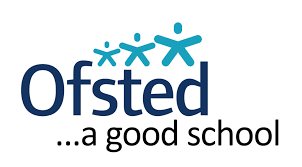••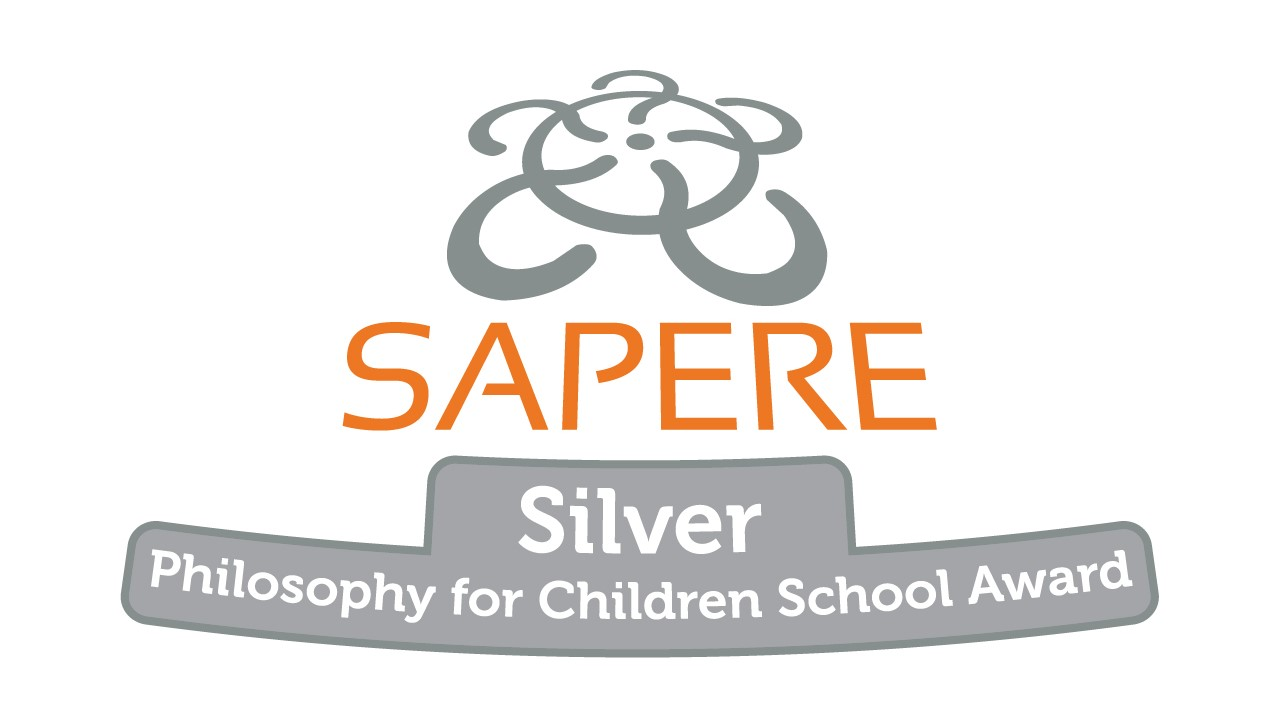•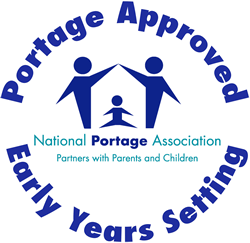•